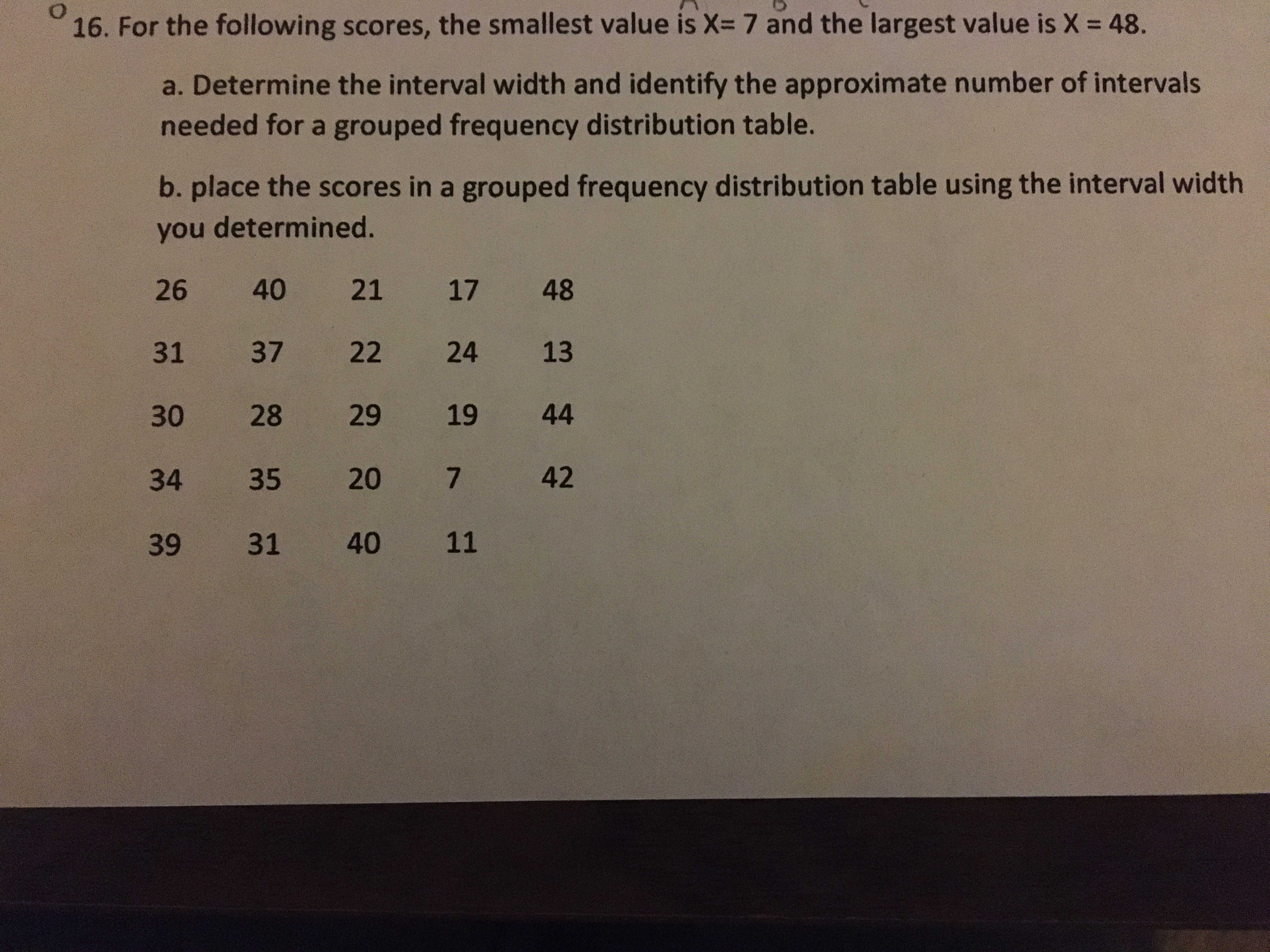# 16. For the following scores, the smallest value is X= 7 and the largest value is X = 48.%3Da. Determine the interval width and identify the approximate number of intervalsneeded for a grouped frequency distribution table.b. place the scores in a grouped frequency distribution table using the interval widthyou determined.2640211748313722241330282919443435204239314011

Question
109 views

help solve the this problemhelp_outlineImage Transcriptionclose16. For the following scores, the smallest value is X= 7 and the largest value is X = 48. %3D a. Determine the interval width and identify the approximate number of intervals needed for a grouped frequency distribution table. b. place the scores in a grouped frequency distribution table using the interval width you determined. 26 40 21 17 48 31 37 22 24 13 30 28 29 19 44 34 35 20 42 39 31 40 11 fullscreen
check_circle

Step 1

a)

The given is ranges from 7 to 48. Since, the number of class interval for the data should be minimum...

### Want to see the full answer?

See Solution

#### Want to see this answer and more?

Solutions are written by subject experts who are available 24/7. Questions are typically answered within 1 hour.*

See Solution
*Response times may vary by subject and question.
Tagged in

### Other